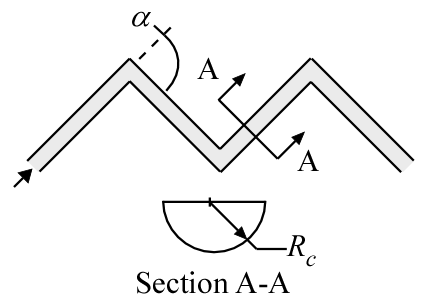Contents - Index

ZigZag_localProcedure ZigZag_local(Fluid\$, T, P, m_dot, R_c, alpha: h, dPdx, Nusselt,  f, Re) returns the local (averaged over a unit cell) heat transfer coefficient and pressure gradient associated with flow through a zig-zag channel.  The procedure also provides the local Nusselt number, local friction factor, and Reynolds number (entrance effects are not included - these are local in that they are at a particular location)

Inputs:

Fluid\$ can be any fluid in the EES database.  The fluid can be an ideal gas or a real fluid.

T - the bulk temperature of the fluid in  [C], [K], [F], or [R].

P -  pressure in [Pa], [kPa], [bar], [MPa], [atm], or [psia].

m_dot  -  mass flow rate in [kg/s], or [lbm/hr]

R_c - channel radius [m] or [ft]

alpha - angle [degree] or [radian]

Outputs:

h  -  local heat transfer coefficient in [W/m^2-K] or [Btu/hr-ft^2-R]

dPdx -  local pressure gradient.  [(Pa, kPa, bar,  or atm)/m] or  [(psi or atm)/ft] depending on EES pressure unit setting

Nusselt - local Nusselt number

f - local friction factor

Re - Reynolds number based on properties evaluated at the bulk average temperature

Notes:

This procedure calls ZigZag_ND_local to obtain the Nusselt numbers and friction factor using the correlation from Moisseytsev et al. (2020)

Example:

\$UnitSystem SI Mass J K Pa Radian

Fluid\$='CarbonDioxide'

T=750 [K]

P=10e6 [Pa]

R_c=2 [mm]*convert(mm,m)

m_dot=0.001 [kg/s]

Call zigzag_local(Fluid\$, T, P, m_dot, R_c, alpha: h, dPdx, Nusselt,  f, Re)

{Solution:

h = 1056 [W/m^2-K]

dPdx = 6243 [Pa/m]

Nusselt = 46.81

f = 0.08435

Re = 11425

}